# Motion With Graphs with Examples

Article Index
Motion With Graphs
Motion With Graphs (Continue)
All Pages

DESCRIBING MOTION WITH GRAPHS

Position vs. Time Graphs:

1. Graphs are commonly used in physics. They give us much information about the concepts and we can infer many things. Let’s talk about this position vs. time graph. As you see on the graph, X axis shows us time and Y axis shows position. We observe that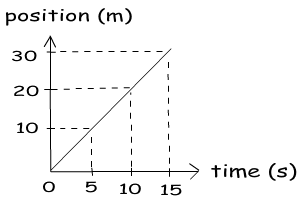position is linearly increasing in positive direction with the time. We understand from this linear increasing, our velocity is constant. If it was not constant we would see a curved line in our graph. Now, we use this graph and make some calculations.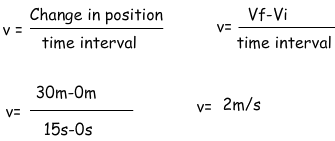From the given graph we calculate velocity; there is another way of this calculation. We just look at the slope of the graph and find the velocity. What we mean by slope is;which is the equation we use in calculation of the velocity.

2. Position is increasing positive direction.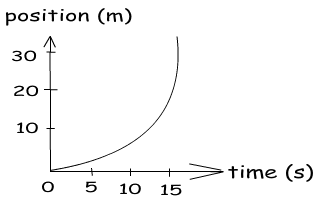In this graph our velocity is changing. As a result of this change graph has curved line not linear. So position does not increase linearly. We can also find the velocity of the object from this graph. We should first find the slope of the curve and calculate the velocity.

Example Using the given graph find the velocity of the object in intervals (1s – 3s) and (3s – 5s).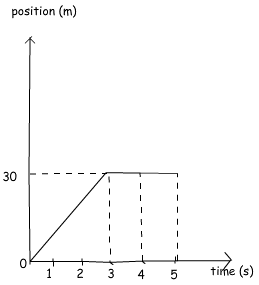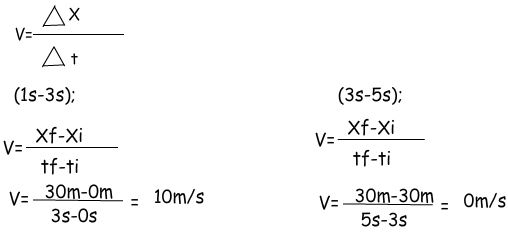In graph problems you should be careful while reading it. For example, in this example in the interval (3s-5s) position does not change. You can easily see it from the graph, but I want to show the calculation of this and it gives us same result. If there is no change in position then there is no velocity or vice versa. You can say more things about the motion of the object by just looking of the graph. The important thing is that you must know the relations, meaning of the slopes or area of the graphs. We will solve more examples using graph for deep understanding and analyzing the motion from the graphs.

3. This position time graph is an example of increasing position in negative direction. Red line shows nonlinear increasing and black line shows linear increasing. We say that linear increasing in position is the result of constant velocity which means zero acceleration. Moreover, nonlinear increase in the position is the result of changing velocity and it shows there is a nonzero acceleration.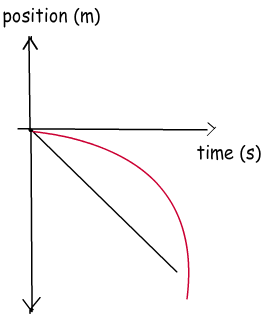4. Until now we saw graphics including speeding up motion in positive and negative directions. Now we talk about a little bit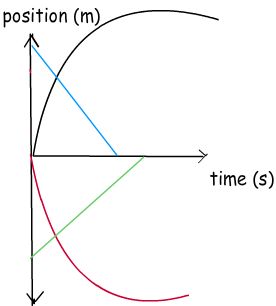slowing down object’s graphs. For example, in this given graph, our position is decreasing in different directions. Red line shows the position of the object which is slowing down in negative direction and black line shows the position of the object slowing down in positive direction. However, the blue and green lines show the linear decrease in position in positive and negative directions.

We have seen various type of position vs. time graphs. I think they will help you in solving problems. It is really easy, you should just keep in mind that “slope shows the velocity”.

Related